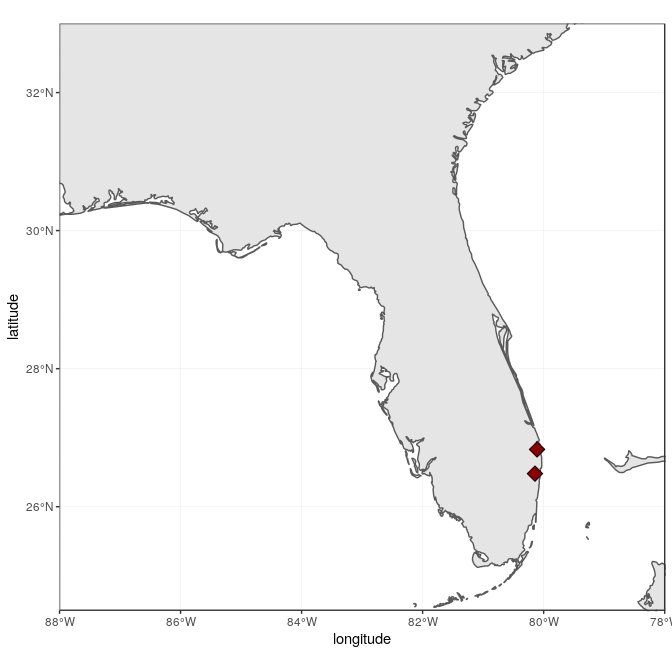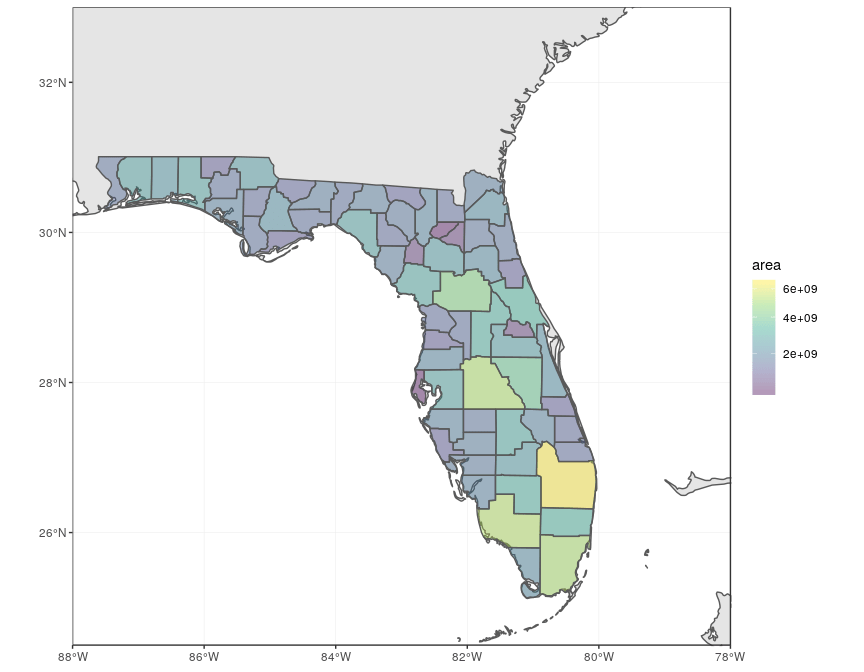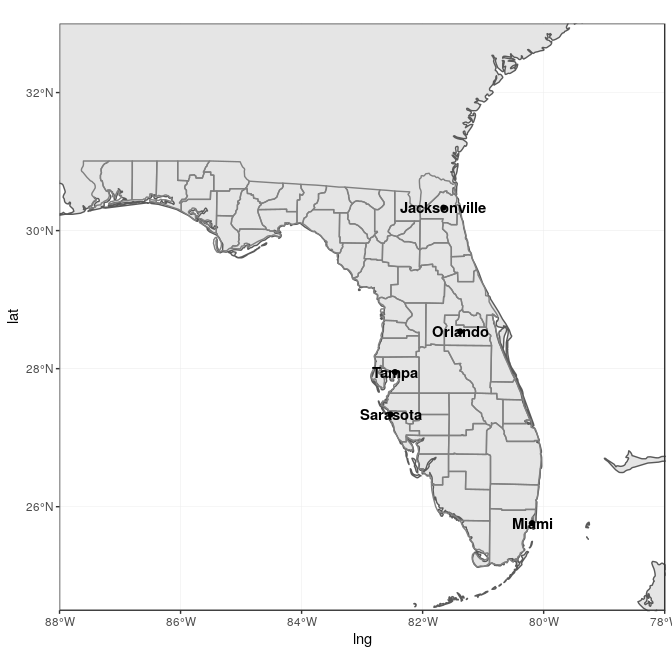Want to share your content on R-bloggers? click here if you have a blog, or here if you don't.

view raw Rmd

This tutorial is the second part in a series of three:

In the previous part, we presented general concepts with a map with little information (country borders only). The modular approach of `ggplot2` allows to successively add additional layers, for instance study sites or administrative delineations, as will be illustrated in this part.

## Getting started

Many R packages are available from CRAN, the Comprehensive R Archive Network, which is the primary repository of R packages. The full list of packages necessary for this series of tutorials can be installed with:

```install.packages(c("cowplot", "googleway", "ggplot2", "ggrepel",
"ggspatial", "libwgeom", "sf", "rworldmap", "rworldxtra"))
```

We start by loading the basic packages necessary for all maps, i.e. `ggplot2` and `sf`. We also suggest to use the classic dark-on-light theme for `ggplot2` (`theme_bw`), which is more appropriate for maps:

```library("ggplot2")
theme_set(theme_bw())
library("sf")
```

The package `rworldmap` provides a map of countries of the entire world; a map with higher resolution is available in the package `rworldxtra`. We use the function `getMap` to extract the world map (the resolution can be set to `"low"`, if preferred):

```library("rworldmap")
library("rworldxtra")
world <- getMap(resolution = "high")
class(world)

##  "SpatialPolygonsDataFrame"
## attr(,"package")
##  "sp"
```

The world map is available as a `SpatialPolygonsDataFrame` from the package `sp`; we thus convert it to a simple feature using `st_as_sf` from package `sf`:

```world <- st_as_sf(world)
class(world)

##  "sf"         "data.frame"
```

## Field sites (point data)

We start by defining two study sites, according to their longitude and latitude, stored in a regular `data.frame`:

```(sites <- data.frame(longitude = c(-80.144005, -80.109), latitude = c(26.479005,
26.83)))

##   longitude latitude
## 1 -80.14401 26.47901
## 2 -80.10900 26.83000
```

The quickest way to add point coordinates is with the general-purpose function `geom_point`, which works on any X/Y coordinates, of regular data points (i.e. not geographic). As such, we can adjust all characteristics of points (e.g. color of the outline and the filling, shape, size, etc.), for all points, or using grouping from the data (i.e defining their “aesthetics”). In this example, we add the two points as diamonds (`shape = 23`), filled in dark red (`fill = "darkred"`) and of bigger size (`size = 4`):

```ggplot(data = world) +
geom_sf() +
geom_point(data = sites, aes(x = longitude, y = latitude), size = 4,
shape = 23, fill = "darkred") +
coord_sf(xlim = c(-88, -78), ylim = c(24.5, 33), expand = FALSE)
```A better, more flexible alternative is to use the power of `sf`: Converting the data frame to a `sf` object allows to rely on `sf` to handle on the fly the coordinate system (both projection and extent), which can be very useful if the two objects (here world map, and sites) are not in the same projection. To achieve the same result, the projection (here WGS84, which is the CRS code #4326) has to be a priori defined in the `sf` object:

```(sites <- st_as_sf(sites, coords = c("longitude", "latitude"),
crs = 4326, agr = "constant"))

## Simple feature collection with 2 features and 0 fields
## geometry type:  POINT
## dimension:      XY
## bbox:           xmin: -80.14401 ymin: 26.479 xmax: -80.109 ymax: 26.83
## epsg (SRID):    4326
## proj4string:    +proj=longlat +datum=WGS84 +no_defs
##                     geometry
## 1 POINT (-80.14401 26.47901)
## 2      POINT (-80.109 26.83)

ggplot(data = world) +
geom_sf() +
geom_sf(data = sites, size = 4, shape = 23, fill = "darkred") +
coord_sf(xlim = c(-88, -78), ylim = c(24.5, 33), expand = FALSE)
```Note that `coord_sf` has to be called after all `geom_sf` calls, as to supersede any former input.

## States (polygon data)

It would be informative to add finer administrative information on top of the previous map, starting with state borders and names. The package `maps` (which is automatically installed and loaded with `ggplot2`) provides maps of the USA, with state and county borders, that can be retrieved and converted as `sf` objects:

```library("maps")
states <- st_as_sf(map("state", plot = FALSE, fill = TRUE))

## Simple feature collection with 6 features and 1 field
## geometry type:  MULTIPOLYGON
## dimension:      XY
## bbox:           xmin: -124.3834 ymin: 30.24071 xmax: -71.78015 ymax: 42.04937
## epsg (SRID):    4326
## proj4string:    +proj=longlat +datum=WGS84 +no_defs
##                         geometry          ID
## 1 MULTIPOLYGON (((-87.46201 3...     alabama
## 2 MULTIPOLYGON (((-114.6374 3...     arizona
## 3 MULTIPOLYGON (((-94.05103 3...    arkansas
## 4 MULTIPOLYGON (((-120.006 42...  california
## 5 MULTIPOLYGON (((-102.0552 4...    colorado
## 6 MULTIPOLYGON (((-73.49902 4... connecticut
```

State names are part of this data, as the `ID` variable. A simple (but not necessarily optimal) way to add state name is to compute the centroid of each state polygon as the coordinates where to draw their names. Centroids are computed with the function `st_centroid`, their coordinates extracted with `st_coordinates`, both from the package `sf`, and attached to the state object:

```states <- cbind(states, st_coordinates(st_centroid(states)))
```

Note the warning, which basically says that centroid coordinates using longitude/latitude data (i.e. WGS84) are not exact, which is perfectly fine for our drawing purposes. State names, which are not capitalized in the data from `maps`, can be changed to title case using the function `toTitleCase` from the package `tools`:

```library("tools")
states\$ID <- toTitleCase(states\$ID)

## Simple feature collection with 6 features and 3 fields
## geometry type:  MULTIPOLYGON
## dimension:      XY
## bbox:           xmin: -124.3834 ymin: 30.24071 xmax: -71.78015 ymax: 42.04937
## epsg (SRID):    4326
## proj4string:    +proj=longlat +datum=WGS84 +no_defs
##            ID          X        Y                       geometry
## 1     Alabama  -86.83042 32.80316 MULTIPOLYGON (((-87.46201 3...
## 2     Arizona -111.66786 34.30060 MULTIPOLYGON (((-114.6374 3...
## 3    Arkansas  -92.44013 34.90418 MULTIPOLYGON (((-94.05103 3...
## 4  California -119.60154 37.26901 MULTIPOLYGON (((-120.006 42...
## 5    Colorado -105.55251 38.99797 MULTIPOLYGON (((-102.0552 4...
## 6 Connecticut  -72.72598 41.62566 MULTIPOLYGON (((-73.49902 4...
```

To continue adding to the map, state data is directly plotted as an additional `sf` layer using `geom_sf`. In addition, state names will be added using `geom_text`, declaring coordinates on the X-axis and Y-axis, as well as the label (from `ID`), and a relatively big font size.

```ggplot(data = world) +
geom_sf() +
geom_sf(data = states, fill = NA) +
geom_text(data = states, aes(X, Y, label = ID), size = 5) +
coord_sf(xlim = c(-88, -78), ylim = c(24.5, 33), expand = FALSE)
```We can move the state names slightly to be able to read better “South Carolina” and “Florida”. For this, we create a new variable `nudge_y`, which is -1 for all states (moved slightly South), 0.5 for Florida (moved slightly North), and -1.5 for South Carolina (moved further South):

```states\$nudge_y <- -1
states\$nudge_y[states\$ID == "Florida"] <- 0.5
states\$nudge_y[states\$ID == "South Carolina"] <- -1.5
```

To improve readability, we also draw a rectangle behind the state name, using the function `geom_label` instead of `geom_text`, and plot the map again.

```ggplot(data = world) +
geom_sf() +
geom_sf(data = states, fill = NA) +
geom_label(data = states, aes(X, Y, label = ID), size = 5, fontface = "bold",
nudge_y = states\$nudge_y) +
coord_sf(xlim = c(-88, -78), ylim = c(24.5, 33), expand = FALSE)
```## Counties (polygon data)

County data are also available from the package `maps`, and can be retrieved with the same approach as for state data. This time, only counties from Florida are retained, and we compute their area using `st_area` from the package `sf`:

```counties <- st_as_sf(map("county", plot = FALSE, fill = TRUE))
counties <- subset(counties, grepl("florida", counties\$ID))
counties\$area <- as.numeric(st_area(counties))

## Simple feature collection with 6 features and 2 fields
## geometry type:  MULTIPOLYGON
## dimension:      XY
## bbox:           xmin: -85.98951 ymin: 25.94926 xmax: -80.08804 ymax: 30.57303
## epsg (SRID):    4326
## proj4string:    +proj=longlat +datum=WGS84 +no_defs
##                           geometry               ID       area
## 292 MULTIPOLYGON (((-82.66062 2...  florida,alachua 2498863359
## 293 MULTIPOLYGON (((-82.04182 3...    florida,baker 1542466064
## 294 MULTIPOLYGON (((-85.40509 3...      florida,bay 1946587533
## 295 MULTIPOLYGON (((-82.4257 29... florida,bradford  818898090
## 296 MULTIPOLYGON (((-80.94747 2...  florida,brevard 2189682999
## 297 MULTIPOLYGON (((-80.89018 2...  florida,broward 3167386973
```

County lines can now be added in a very simple way, using a gray outline:

```ggplot(data = world) +
geom_sf() +
geom_sf(data = counties, fill = NA, color = gray(.5)) +
coord_sf(xlim = c(-88, -78), ylim = c(24.5, 33), expand = FALSE)
```We can also fill in the county using their area to visually identify the largest counties. For this, we use the “viridis” colorblind-friendly palette, with some transparency:

```ggplot(data = world) +
geom_sf() +
geom_sf(data = counties, aes(fill = area)) +
scale_fill_viridis_c(trans = "sqrt", alpha = .4) +
coord_sf(xlim = c(-88, -78), ylim = c(24.5, 33), expand = FALSE)
```## Cities (point data)

To make a more complete map of Florida, main cities will be added to the map. We first prepare a data frame with the five largest cities in the state of Florida, and their geographic coordinates:

```flcities <- data.frame(state = rep("Florida", 5), city = c("Miami",
"Tampa", "Orlando", "Jacksonville", "Sarasota"), lat = c(25.7616798,
27.950575, 28.5383355, 30.3321838, 27.3364347), lng = c(-80.1917902,
-82.4571776, -81.3792365, -81.655651, -82.5306527))
```

Instead of looking up coordinates manually, the package `googleway` provides a function `google_geocode`, which allows to retrieve geographic coordinates for any address, using the Google Maps API. Unfortunately, this requires a valid Google API key (follow instructions here to get a key, which needs to include “Places” for geocoding). Once you have your API key, you can run the following code to automatically retrieve geographic coordinates of the five cities:

```library("googleway")
key <- "put_your_google_api_key_here" # real key needed
flcities <- data.frame(state = rep("Florida", 5), city = c("Miami",
"Tampa", "Orlando", "Jacksonville", "Sarasota"))
coords <- apply(flcities, 1, function(x) {
key = key)
})
flcities <- cbind(flcities, do.call(rbind, lapply(coords, geocode_coordinates)))
```

We can now convert the data frame with coordinates to `sf` format:

```(flcities <- st_as_sf(flcities, coords = c("lng", "lat"), remove = FALSE,
crs = 4326, agr = "constant"))

## Simple feature collection with 5 features and 4 fields
## Attribute-geometry relationship: 4 constant, 0 aggregate, 0 identity
## geometry type:  POINT
## dimension:      XY
## bbox:           xmin: -82.53065 ymin: 25.76168 xmax: -80.19179 ymax: 30.33218
## epsg (SRID):    4326
## proj4string:    +proj=longlat +datum=WGS84 +no_defs
##     state         city      lat       lng                   geometry
## 1 Florida        Miami 25.76168 -80.19179 POINT (-80.19179 25.76168)
## 2 Florida        Tampa 27.95058 -82.45718 POINT (-82.45718 27.95058)
## 3 Florida      Orlando 28.53834 -81.37924 POINT (-81.37924 28.53834)
## 4 Florida Jacksonville 30.33218 -81.65565 POINT (-81.65565 30.33218)
## 5 Florida     Sarasota 27.33643 -82.53065 POINT (-82.53065 27.33643)
```

We add both city locations and names on the map:

```ggplot(data = world) +
geom_sf() +
geom_sf(data = counties, fill = NA, color = gray(.5)) +
geom_sf(data = flcities) +
geom_text(data = flcities, aes(x = lng, y = lat, label = city),
size = 3.9, col = "black", fontface = "bold") +
coord_sf(xlim = c(-88, -78), ylim = c(24.5, 33), expand = FALSE)
```This is not really satisfactory, as the names overlap on the points, and they are not easy to read on the grey background. The package `ggrepel` offers a very flexible approach to deal with label placement (with `geom_text_repel` and `geom_label_repel`), including automated movement of labels in case of overlap. We use it here to “nudge” the labels away from land into the see, and connect them to the city locations:

```library("ggrepel")
ggplot(data = world) +
geom_sf() +
geom_sf(data = counties, fill = NA, color = gray(.5)) +
geom_sf(data = flcities) +
geom_text_repel(data = flcities, aes(x = lng, y = lat, label = city),
fontface = "bold", nudge_x = c(1, -1.5, 2, 2, -1), nudge_y = c(0.25,
-0.25, 0.5, 0.5, -0.5)) +
coord_sf(xlim = c(-88, -78), ylim = c(24.5, 33), expand = FALSE)
```# Final map

For the final map, we put everything together, having a general background map based on the world map, with state and county delineations, state labels, main city names and locations, as well as a theme adjusted with titles, subtitles, axis labels, and a scale bar:

```library("ggspatial")
ggplot(data = world) +
geom_sf(fill = "antiquewhite1") +
geom_sf(data = counties, aes(fill = area)) +
geom_sf(data = states, fill = NA) +
geom_sf(data = sites, size = 4, shape = 23, fill = "darkred") +
geom_sf(data = flcities) +
geom_text_repel(data = flcities, aes(x = lng, y = lat, label = city),
fontface = "bold", nudge_x = c(1, -1.5, 2, 2, -1), nudge_y = c(0.25,
-0.25, 0.5, 0.5, -0.5)) +
geom_label(data = states, aes(X, Y, label = ID), size = 5, fontface = "bold",
nudge_y = states\$nudge_y) +
scale_fill_viridis_c(trans = "sqrt", alpha = .4) +
annotation_scale(location = "bl", width_hint = 0.4) +
annotation_north_arrow(location = "bl", which_north = "true",
style = north_arrow_fancy_orienteering) +
coord_sf(xlim = c(-88, -78), ylim = c(24.5, 33), expand = FALSE) +
xlab("Longitude") + ylab("Latitude") +
ggtitle("Observation Sites", subtitle = "(2 sites in Palm Beach County, Florida)") +
theme(panel.grid.major = element_line(color = gray(0.5), linetype = "dashed",
size = 0.5), panel.background = element_rect(fill = "aliceblue"))
```This example fully demonstrates that adding layers on `ggplot2` is relatively straightforward, as long as the data is properly stored in an `sf` object. Adding additional layers would simply follow the same logic, with additional calls to `geom_sf` at the right place in the `ggplot2` sequence.